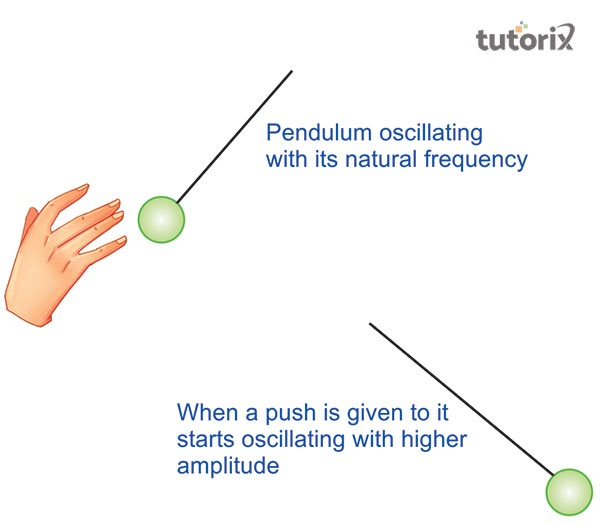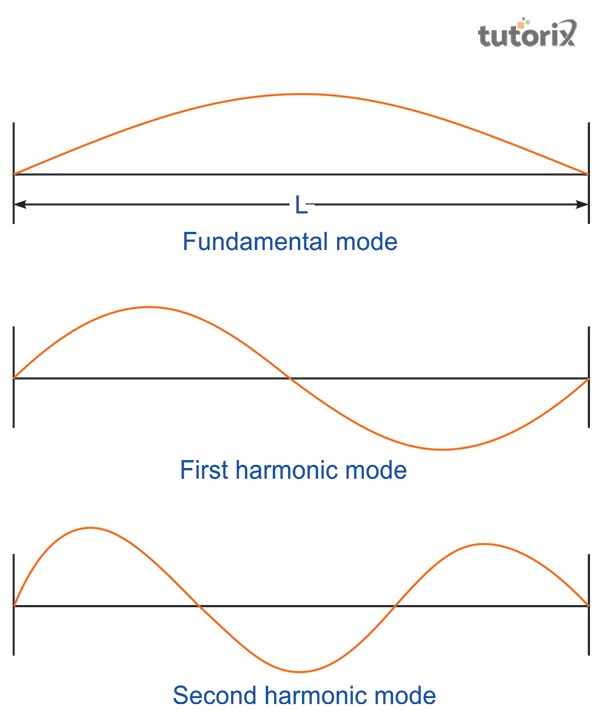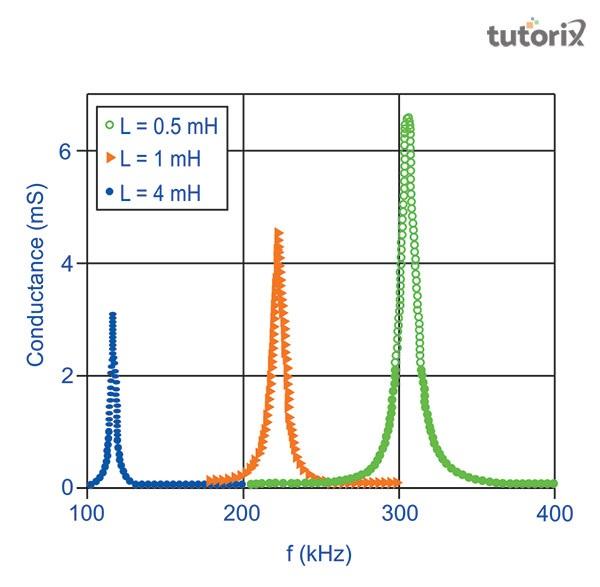# Resonance

## Introduction

In introducing this it needs to be understood that the phenomenon of resonance is used in determining the unknown frequency. It is a special phenomenon, which is used in our daily lives, majorly in the field of music. It is sometimes used to understand several musical instruments, and sometimes used to increase the extent of intensity of sounds' ' in several instruments of music. However, to tune the radio receiver, the phenomenon of resonance is used.

## Definition of Resonance

Resonance is a peculiar phenomenon, where an external force or any other forces of vibrating substances tends to exert pressure on another system. These further results in vibrating to a much greater extent with relatively high amplitude that is conducted under a specified frequency associated with operations (Jones & Bradshaw, 2019). This condition occurs when the two sound systems tend to have the matching frequency which results in an increase in the notion of amplitude.

## Principle of resonance

In the case of the phenomenon of resonance, if the frequency of resonance is having linear oscillations $\Omega$, then it is said to have been running from a source having a frequency, $\omega$ , then the intensity of oscillations can be defined as $\mathrm{f\:=\:12\pi\Gamma}$ (Sciencing.com, 2022). In this formula, $\mathrm{\Gamma}$ is detonated as, a situation for damping, when the system is known to be Linewidth.

## Examples of Resonance

Several examples of the phenomenon of resonance are evidenced in our daily lives, such as, in a vibration created by a swing, in the operation of televisions and radio. In the laser, which is known to be an electromagnetic wave the phenomenon of resonance is being used. Furthermore, in several musical instruments, the notion of resonance is observed (Quantamagazine.org, 2022). At times the crystal wineglass, shatters as it comes into close contact with the tones of music with a particular pitch.Figure 1: Example of resonance

## Mathematical representation of Resonance

The mathematical representation of resonance can be defined in this way-

$\mathrm{v\:=\:\lambda f}$

This formula presents the frequency of resonance of a particular wave. In this formula, v means the wave velocity, and λ means the distance of wavelength. The meaning of this formula is that wave velocity is equal to the distance of the wavelength (Bien et al. 2018). It is multiplied by the frequency f resonance. It mainly refers to the state or equity of being resonant. Here resonance is the concept of the ability of the system to move the electrons within the system.

## Different types of resonances

### Acoustic resonance

Acoustic resonance is an essential type of resonance and it is seen in the musical instruments. It is because most of the resonators are present in the musical instruments. It includes the body and strings of a violin and it is also seen in the length of a tube (Ferreira et al. 2018). Besides these factors, this type of resonance also works for hearing too. In this resonance, the sound waves are watched to their own natural frequency of vibration. This type of resonance is mainly used for the absorption of sound in a specific range.

### Mechanical resonance

In the system of mechanics, Mechanical Resonance is a type of resonance that responded at greater amplitude. This process happens at the time of natural frequency of the system (Bien et al. 2018). This is also called the resonant frequency. Mechanical resonance mainly refers to the tendency that responds at greater amplitude.Figure 2: Mechanical resonance

This process occurs when the frequency matches the frequency of vibration. The most common example of this resonance is the breaking of glass of an opera singer through his voice with the right note. It is seen that when the frequency of a sound is aligned with the resonant frequency of the material, then this resonance happens.

### Electrical resonanceFigure 3: Variation of electrical resonance frequency with inductance

Electrical resonance is present in an electric circuit and this circuit occurs when the capacitive reactance and inductive reactance remain equal in the magnitude. These types of resonance occur in the electric field when the output and input of the electric circuit are almost zero (Researchgate.net, 2018). In this situation, the transfer function of the circuit is almost one. This type of resonance occurs in the electric circuit and at this time the series impedance remains minimum and parallel impedance remains maximum.

## Conclusion

Resonance is the system having a nature of oscillating with the larger amplitudes. This system has the frequency and this is the condition in which the frequency becomes highest and this frequency is called the resonant frequency. This type of frequency is associated with all kinds of waves or vibrations. This type of wave can mainly happen in the Electromagnetic, Sound, and Mechanical wave. The particles of different types of resonant increase the importance of the part of resonant. The important thing that is needed for resonant is the object or the system.

## FAQs

Q1. What are the basic causes of resonance?

Ans. The basic causes for the phenomenon of occurrences of resonance, can be determined by matching vibrations to several other objects that tend to increase the rate of amplitudes of the oscillated object.

Q2. What is determined by the resonant frequency?

Ans. The term resonant frequency is determined by the natural frequency, within a medium, where it vibrates with the highest amount of amplitudes.

Q3. Why is it said that due to the resonance the bridge may collapse?

Ans. It is said that a group of soldiers marching towards the bridge may be the reason for the crushing of the bridge. This is because, due to the notion of rhythmic marching of the soldiers, extreme vibrations in being set to that of the natural frequency exhibited by the bridge. Therefore, with the synchronized footsteps, the amplitude may increase so high that the bridge may tend to collapse. For this reason, while crossing a bridge the soldiers are instructed not to march.